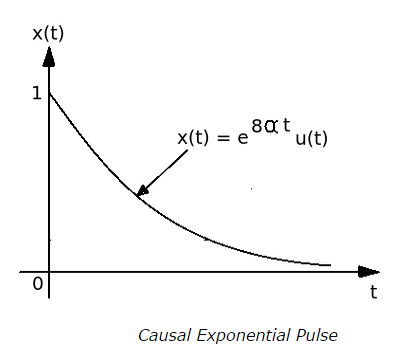# Correlation and Spectral Density - MCQs with answers

## Correlation and Spectral Density - MCQs with answers

1. Correlogram is a graph of ______

a. Amplitude of one signal plotted against the amplitude of another signal

b. Frequency of one signal plotted against the frequency of another signal

c. Amplitude of one signal plotted against the frequency of another signal

d. Frequency of one signal plotted against the time period of another signal

ANSWER: a. Amplitude of one signal plotted against the amplitude of another signal

2. Which theorem states that the total average power of a periodic signal is equal to the sum of average powers of the individual fourier coefficients?

a. Parseval's Theorem
b. Rayleigh's Theorem
c. Both a & b
d. None of the above

3. According to Rayleigh's theorem, it becomes possible to determine the energy of a signal by________

a. Estimating the area under the square root of its amplitude spectrum

b. Estimating the area under the square of its amplitude spectrum

c. Estimating the area under the one-fourth power of its amplitude spectrum

d. Estimating the area exactly half as that of its amplitude spectrum

ANSWER: b. Estimating the area under the square of its amplitude spectrum

4. What would be the value of normalized energy for the causal exponential pulse shown below?a. 8α

b. 1 / 8α

c. -1 / 8α

d. -8α

5. What does the spectral density function of any signal specify?

a. Distribution of energy or power
b. Consumption of energy or power
c. Conservation of energy or power
d. Generation of energy or power

ANSWER: a. Distribution of energy or power

6. Which among the below mentioned transform pairs is/are formed between the auto-correlation function and the energy spectral density, in accordance to the property of Energy Spectral Density (ESD)?

a. Laplace Transform
b. Z-transform
c. Fourier Transform
d. All of the above

7. The ESD of a real valued energy signal is always _________

a. An even (symmetric) function of frequency
b. An odd (non-symmetric) function of frequency
c. A function that is odd and half-wave symmetric
d. None of the above

ANSWER: a. An even (symmetric) function of frequency

8. Which among the below mentioned assertions is /are correct?

A. Greater the value of correlation function, higher is the similarity level between two signals

B. Greater the value of correlation function, lower is the similarity level between two signals

C. Lesser the value of correlation function, higher is the similarity level between two signals

D. Lesser the value of correlation function, lower is the similarity level between two signals

a. Only C
b. Only B
c. A & D
d. B & C

9. Which function has a provision of determining the similarity between the signal and its delayed version?

a. Auto-correlation Function
b. Cross-correlation Function
c. Both a & b
d. None of the above

10. Which property is exhibited by the auto-correlation function of a complex valued signal?

a. Commutative property
b. Distributive property
c. Conjugate property
d. Associative property

11. Where does the maximum value of auto-correlation function of a power signal occur?

a. At origin
b. At extremities
c. At unity
d. At infinity